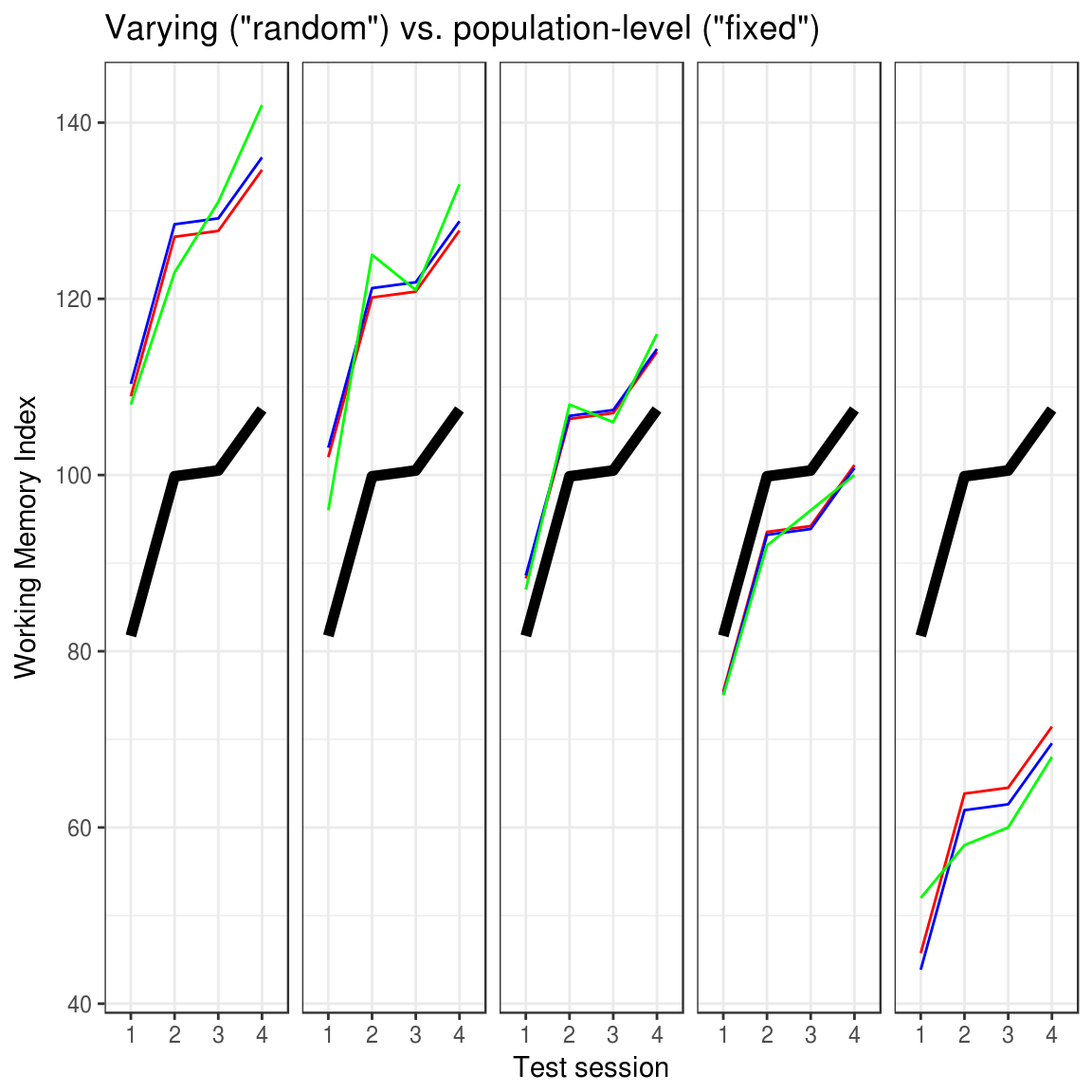This notebook shows the code used to generate the figure that accompanies the post Let’s rename “fixed” to “Population-level” and “Random” to “Varying”. And then some.

Let’s first load some data. This is from our Brain paper, which I tend to use. Each patient is tested four times on a Working Memory Index (WMI) in the course of a surprisingly effective treatment. Let’s load the data:

# Fit models

# Process data: make factors and take subset
D$session = factor(D$session)
D$id = factor(D$id)
D = subset(D, group == 'groupA')

Now let’s fit the data with models where subject is modeled as a varying or population-level intercept and add the predictions from those models back into the dataset:

# Fit different models
fit_varying = lme4::lmer(WMI ~ session + (1|id), D)  # Varying subject-intercept
fit_population = lm(WMI ~ session + id, D)  # Population-level subject-intercept
fit_mean = lm(WMI ~ session, D)  # No subject-intercept

# Add model predictions to data
D$pred_varying = predict(fit_varying) # Varying D$pred_population = predict(fit_population)
D$pred_mean = predict(fit_mean) # Plot it # Plot model predictions library(ggplot2) D_plot = subset(D, id %in% c(1, 39, 66, 43, 45)) ggplot(D_plot, aes(x = session, y=WMI, group = 1)) + geom_line(aes(y=pred_mean), color='black', lwd=2) + geom_line(aes(y=pred_varying), color='red') + geom_line(aes(y=pred_population), color='blue') + geom_line(aes(y=WMI), color='green') + #geom_line(color='green') + facet_wrap(~id, ncol=5) + # Styling theme_bw() + theme(strip.background = element_blank(), strip.text.x = element_blank()) + labs(x = 'Test session', y = 'Working Memory Index', title='Varying ("random") vs. population-level ("fixed")') + scale_color_manual(values=c('goat'="#999999", "#E69F00", "#56B4E9"))#scale_fill_discrete(name="Model", # labels=c("Varying ID", "Population ID", "No ID"), # values=c("#999999", "#E69F00", "#56B4E9")) Interesting stuff! Notice how the model with varying subject intercept (red line) is always closer to the population mean (black line) than the model with population-level intercept (blue line). This is shrinkage in action! Also, the population-model fits the data (green line) better than the varying model, showcasing the difference between in-sample modeling (population) and out-of-sample modeling (varying). # Look at parameters If it wasn’t clear from the plots, the models are ALMOST identical. In fact, they are EXACTLY identical with respect to the mean and variance of the estimates for each session which was population-level in both models. This is very reassuring. Note that the difference in the population-level intercepts is purely due to different parameterization of the models. lmer takes the mean of session1 and lm takes the score of session1 for the first participant (see plot). summary(fit_varying)$coefficients
##             Estimate Std. Error  t value
## (Intercept) 81.74074   2.857115 28.60954
## session2    18.11111   1.679957 10.78070
## session3    18.77778   1.679957 11.17754
## session4    25.70370   1.679957 15.30022
head(summary(fit_population)\$coefficients)
##              Estimate Std. Error   t value     Pr(>|t|)
## (Intercept) 110.35185   3.253222 33.920790 1.874048e-48
## session2     18.11111   1.679957 10.780702 4.077081e-17
## session3     18.77778   1.679957 11.177538 7.262397e-18
## session4     25.70370   1.679957 15.300220 3.385067e-25
## id10        -19.75000   4.364655 -4.524985 2.131761e-05
## id17        -30.75000   4.364655 -7.045230 6.460493e-10

… and so